Journal of Multimedia Information System
Korea Multimedia Society
Section D

# Heterogeneous Large-Scale Data Fusion Mechanism of Energy Storage Power Station Based on Neural Network

Yimin Deng1,*, Zhoubo Weng1, Tianlong Zhang1
1State Grid Zhejiang Electric Power Co., Ltd., Hangzhou, China, dengyiming2022@163.com, weng_zbo@126.com, ztl20220602@163.com
*Corresponding Author: Yimin Deng, Address, +86-13588361621, dengyiming2022@163.com

© Copyright 2023 Korea Multimedia Society. This is an Open-Access article distributed under the terms of the Creative Commons Attribution Non-Commercial License (http://creativecommons.org/licenses/by-nc/4.0/) which permits unrestricted non-commercial use, distribution, and reproduction in any medium, provided the original work is properly cited.

Received: May 24, 2023; Revised: Jun 07, 2023; Accepted: Jun 11, 2023

Published Online: Jun 30, 2023

## Abstract

To solve the problems of many automation systems, diverse data standards, and duplication of information content in the current energy storage power station system, and to further improve the freshness, current situation and accuracy of the energy storage power station big data, the heterogeneous large energy storage power station. The fusion of large-scale data has become a general trend. The usual data fusion algorithm is to fuse the features of sensor data in different spaces at the same time. Among them, the deep convolutional neural network (DCNN) has outstanding fusion performance, and realizes the integration of feature extraction, information association, and decision-making judgment by utilizing multiple convolutional layers, pooling layers, and fully connected layers. This paper proposes a data fusion algorithm based on convolutional neural network (AbDCNN-DAE). The algorithm introduces a denoising autoencoder and an attention mechanism, which can fuse data from various aspects to realize the arrangement of disorganized power grid data. The experimental results compared with other methods show that the algorithm proposed in this paper has better performance.

Keywords: Energy Storage Power Station; Heterogeneous Data Fusion; Time Series Data; Deep Convolutional Neural Network

## I. INTRODUCTION

The State Grid Corporation of China has put forward the strategic goal of establishing a world-class energy Internet enterprise in the new era, based on the concept of "three types and two networks". This goal aims to fully leverage the power grid's pivotal role in energy collection, transmission, conversion, and utilization. With the assistance of the energy Internet, various resources will be integrated to establish a robust smart grid and a pervasive power Internet of Things. The concept of "multi-station integration" serves as a fundamental requirement for the construction of the ubiquitous power Internet of Things, and it represents a significant measure to accomplish this strategic objective. "Multi-station integration" is to integrate 5G base stations , Beidou ground enhancement stations , photovoltaic stations , integrated energy stations, etc. It integrates similar sites into one, and finally forms spatial interconnection, logical integration, and horizontal data connection between multiple sites, and cooperates with power grids, financial institutions and other manufacturers to jointly build an ecosystem of the energy industry.

As a critical element of "multi-site integration," energy storage serves as the crucial link connecting multiple sites to achieve energy integration, complementarity, and data integration. In recent years, energy storage power stations have experienced rapid development and are expected to play a pivotal role in future smart energy systems. These power stations effectively function as buffers, aggregators, and stabilizers within the framework of "multi-site integration," ensuring stable and efficient operations across all sites. Ultimately, this facilitates the seamless integration and sharing of diverse energy data. Consequently, data from energy storage power stations serves as the foundation and prerequisite for ensuring successful "multi-station integration." However, with the continuous expansion of energy storage power station development in our country, coupled with advancements in communication technology, Internet technology, and sensors, the quantity and types of energy storage power station data have witnessed an exponential growth trend. The data of energy storage power stations are heterogeneous and large-scale. Massive amounts of data with different forms and structures are collected by a large number of monitoring equipment, analyzed by various monitoring and analysis systems, and computer systems. These data reflect the operating environment and operating status of the smart grid in an all-round way, and have become a very precious resource. How to quickly screen and make full use of the useful information in the massive data stack is a huge challenge facing the current energy storage power station system construction . Therefore, it is a general trend to integrate heterogeneous large-scale data of energy storage power stations.

Data fusion is an emerging technology. It can automatically correlate the collected data and extract features according to a predetermined rule within a complete set of designed algorithm structure, and can more quickly evaluate the status of the research object and process the information of decision-making tasks. To achieve the efficient integration of heterogeneous large-scale data from energy storage power stations, this study presents a novel data fusion mechanism based on convolutional neural networks (CNNs). Specifically, a time series data fusion algorithm is employed to organize and streamline the unstructured data from energy storage power stations. This paper introduces a denoising autoencoder based on a deep convolutional neural network to achieve data denoising reconstruction. In addition, a self-attention mechanism model is introduced to improve the ability to extract key information. The outcome of the experimental evaluations revels that compared with the BPNN, GA-BPNN and DCNN data fusion algorithms, the algorithm in this paper has better fusion performance. This allows for a more detailed and comprehensive analysis of the study subjects.

## II. RELATED WORK

The objective of data fusion technology is to enhance decision-making by integrating multiple sources of information, resulting in more precise and simplified judgments. This involves combining real-time data captured by multiple sensors with information available in related databases to obtain more accurate data. Currently, data fusion technology finds applications across various fields, where it has proven to be highly beneficial, such as face recognition [5-6], disease diagnosis , target tracking , etc. In real life, many data are time series data. However, in addition to the characteristics of large data volume, high dimension, many features, and high noise, time series data [9-10] also has a very important feature, that is, the continuity of data. These continuous data are usually regarded as a whole rather than independent individual. At present, time series data fusion faces two major problems: one is denoising the original data. The data captured by the sensor usually has a lot of ambient noise and equipment noise, and these noises will increase the difficulty of feature extraction, so data denoising is one of the core steps in data fusion. Traditional denoising algorithms mainly include wavelet threshold denoising, empirical mode decomposition (EMD) and so on. Due to the large amount of data and complex structure of multi-source time series data, it is difficult for these methods to obtain stable results. The second is to extract the temporal continuity feature of sequence data from multi-source sensors. The existing feature extraction methods primarily consist of principal component analysis (PCA) and linear discriminant analysis (LDA). However, it is difficult to extract continuous features of time series data.

At present, the time series data fusion algorithms based on neural network include: stacked autoencoder , radial basis function (RBF) neural network , BP neural network [13-14], deep neural network (DNN) , Convolutional Neural Network (CNN) . The literature  shows that the DNN network itself is a fusion structure, which realizes the feature extraction and layer-by-layer fusion of the original data through the connection between the network layers. However, when DNN is used for data fusion, on the one hand, the ability to extract complex features is not high, and on the other hand, it ignores the temporal connection of sequence data. The literature  shows that one-dimensional convolutional neural network can effectively extract the time-related features of time series data. Among them, JING et al.  introduced a deep convolutional neural network (DCNN) architecture. In the DCNN network, the convolutional layer performs feature extraction, and the stacked convolutional layer and pooling layer structure makes the information fused again and builds more complex features, which greatly improves the fusion accuracy. However, the DCNN network ignores the influence of data noise, resulting in low fusion accuracy. The literature [20-21] studies have shown that the denoising auto-encoder (DAE) has a significant effect on the denoising reconstruction of the original data. Attention models have been widely used in machine translation, text classification, recommender systems and other fields. Numerous studies  have shown that attention mechanism models are effective in processing long-range information capture from time series data.

Based on the above discussion, it can be concluded that the original features of the data have a great influence on the learning ability of the deep learning network model, and the introduction of the denoising auto-encoder (DAE) reconstructs the features of the original data to a certain extent. At the same time, considering that the attention mechanism is effective in capturing long-distance information of time series data, this paper proposes a data fusion algorithm based on convolutional neural network, namely AbDCNN-DAE, combining DAE and attention model.

## III. OUR METHOD

3.1. Denoising Autoencoder

Denoising Autoencoder (DAE)  is an artificial neural network approach that can combat raw data contamination, absence, etc. It encodes and decodes the original data by adding Gaussian white noise and uses an unsupervised learning method to train the network to restore the real data, thereby enhancing the robustness of the data. The DAE network uses gradient descent to minimize the cost function:

$minC\left(W,c\right)=\frac{1}{2}{\sum }_{i=1}^{n}{\left({y}^{i}-{z}^{i}\right)}^{2}.$
(1)

to get a set of parameters(W,c)=((W1,c1),(W2,c2)), where the hidden layer is

$h=\delta \left(\stackrel{^}{z}{W}^{1}+{c}^{1}\right).$
(2)

The output layer is

$y=\delta \left(h{W}^{2}+{c}^{2}\right).$
(3)

In the given context, the variables can be defined as follows: i denotes the number of samples, z represents the original sample, represents the sample with added random noise, h denotes the hidden layer, and y represents the output layer. (W1, c1) represents the weight and bias between and h, and W2,c2) represents the weight and bias between h and y. δ =log(exi + 1) represents the softplux activation function.

3.2. 1D-Convolutional Neural Network

The primary architecture of a one-dimensional convolutional neural network (CNN) [24-25] comprises an input layer, a convolutional layer, a pooling layer, a fully connected layer, and an output layer. The convolutional layer is used to extract the abstract features of the data, the pooling layer is used to obtain key information and reduce the dimension, and the fully connected layer is used to synthesize the main information. It is used to aggregate key information and reduce dimensionality. The fully connected layer is employed to integrate and synthesize the primary information.

On the convolution layer, each convolution kernel slides down to perform the convolution operation. The convolutional layer extracts data features through convolutional computation, and obtains more complex abstract features through multiple convolutional layer structures. And the convolution kernel of each convolutional layer is shared weight, which greatly reduces the computational complexity of the neural network. Convolution calculation process:

${x}_{j}^{l}=f\left(\sum _{i\in {M}_{j}}{x}_{i}^{l-1}{k}_{ij}^{l}+{b}_{j}^{l}\right).$
(4)

In this context, the variables can be defined as follows: ${x}_{i}^{l-1}$ represents the input data. f denotes the activation function, and Mj represents the set of input feature maps. ${x}_{j}^{l}$ represents the feature produced by the jth convolution kernel of the lth convolutional layer. ${k}_{ij}^{l}$ and ${b}_{j}^{l}$ represent the weight and bias of the convolution kernel, respectively. In the pooling layer, the key points in the feature map are extracted by the pooling method and the parameter dimension is reduced. Pooling methods are mainly divided into average pooling and maximum pooling: Average pooling computes the output by taking the average value of the data within the pooling window. And maximum pooling takes the largest data in the pooling window as the output. A new feature map is formed by subsampling through a pooling operation. The sampling formula is:

${\stackrel{^}{x}}_{j}^{l}=f\left({\beta }_{j}^{l}down\left({x}_{j}^{l}\right)+{b}_{j}^{l}\right).$
(5)

Among them, ${x}_{j}^{l}$ represents the input, ${\beta }_{j}^{l}$ represents the multiplicative bias, ${b}_{j}^{l}$ represents the additive bias, down() represents the downsampling function, and ${\stackrel{^}{x}}_{j}^{l}$ represents the feature map of the jth convolution kernel of the lth pooling layer.

In the fully connected layer, the feature map can be transformed into a one-dimensional vector, effectively enhancing the characteristics of the original signal, reducing the data dimensionality, and preserving the essential information. Increasing the depth of the fully connected layer can effectively enhance the model's performance and capabilities. At the same time, dropout regularization method is used to prevent overfitting. The output layer utilizes the softmax function to make the final decision (the output layer can be adjusted according to different problems to be solved). The output layer has q possible results.

The Adam algorithm has the advantages of the AdaGrad algorithm and RMSProp, and can adapt to different problems in practice, so this paper uses the Adam optimization algorithm to train the network. The network training method adopts mini-batch training and uses the cross-entropy loss function. It is defined as:

$\text{L}=-\frac{1}{B}\left(Ylg\left({y}_{__}\right)\right),$
(6)

where B, Y, y_ are the mini-batch size, true value and predicted value, respectively.

3.3. Attention Mechanism

Ref.  gave a general framework for the attention mechanism model. The idea of this model comes from the difference in human attention distribution when observing things, and its mathematical essence is weighted summation. The attention mechanism model first uses the dot product as the similarity function to obtain the weight value of the sequence. Afterward, the softmax function is applied to normalize the weights. Finally, the normalized weight matrix is multiplied by the sequence matrix. The calculation formula is:

$\text{Attention}\left(\text{Q},\text{K},\text{V}\right)=\text{softmax}\left(\frac{Q{K}^{T}}{\sqrt{{d}_{k}}}\right)\text{V}.$
(7)

Among them, Q∈Rn×dk, K∈Rm×dk, V∈Rm×dv, Q is the query (query), representing the target sequence value; K, V are the key (key), value (value), respectively, is a set of keys Value pair, representing the key-value pair in the intermediate state of the sequence; by calculating the similarity of Q and K, the weight matrix is obtained; dk is the scale factor; when Q=K=V, it is the self-attention mechanism, which is used to obtain the internal global information.

3.4. The Structure of AbDCNN-DAE Model

The AbDCNN-DAE model primarily consists of two components: the first component employs a denoising auto-encoder (DAE) to denoise and reconstruct the original data, resulting in more resilient and robust data. The second is to add a self-attention mechanism module (referred to as Attention-CNN) on the basis of DCNN to capture the long-distance dependencies of time series data. The original features of the data have a great impact on the performance of deep learning networks, and denoising encoders have good performance in data denoising restoration. Thus, the raw data is first denoised and reconstructed through the DAE network to obtain robust data; then the DAE-processed multi-sensor data is fed into the Attention-CNN model. In the Attention-CNN model, the local features of the time series data are extracted by one-dimensional convolution operation, and then the key information is extracted through the pooling layer. However, the convolution kernel can only extract local information, and it is difficult to obtain long-distance information. Therefore, this paper introduces a self-attention mechanism model to make up for the lack of long- and short-distance dependence of the captured sequence. Therefore, this paper proposes a new algorithm Attention-based DCNN with DAE (AbDCNN-DAE) combining DAE and DCNN network with attention mechanism module. Fig. 1 depicts the structure of the AbDCNN-DAE model.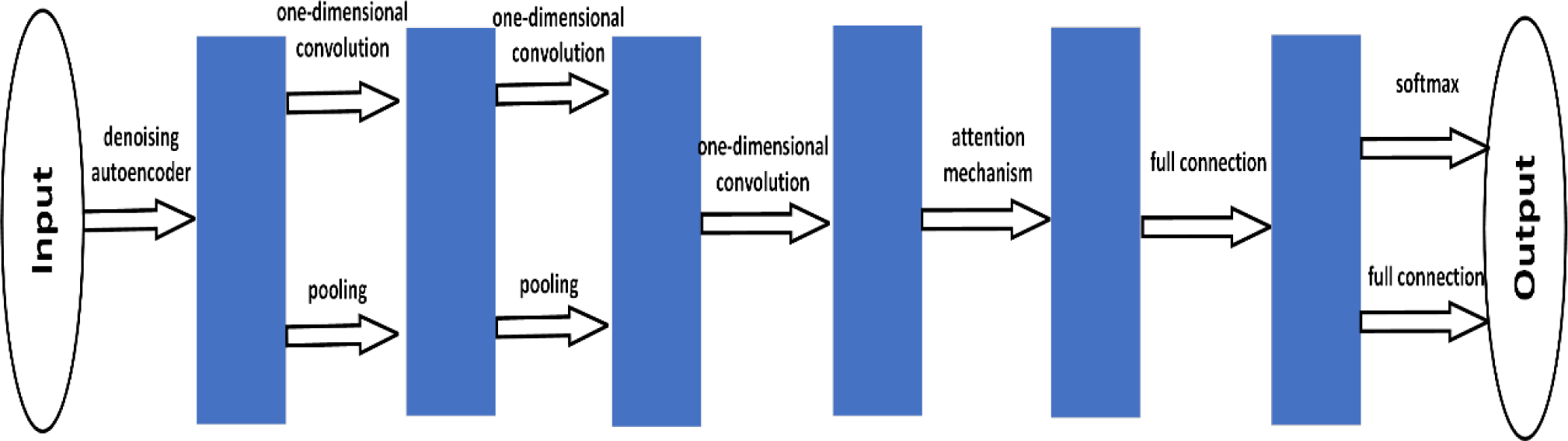Fig. 1. Structure of our model.
3.5. AbDCNN-DAE Model Parameter Settings

The AbDCNN-DAE algorithm includes the DAE model and the Attention-CNN model. The DAE model encompasses an input layer, a hidden layer, and an output layer. In our work, we set the learning rate as 0.001, the training times as 20 and the batch size as 10. On the other hand, the Attention-CNN model is composed of 3 convolutional layers, 2 pooling layers, 1 attention mechanism module, 1 fully connected layer, and 1 fully connected layer with softmax regression. In the Attention-CNN model, the learning rate is set to 0.001, the batch size is 100, and the number of iterations for all samples is established as 200. In our work, the adaptive moment estimation algorithm is selected as the optimization algorithm.

## IV. EXPERIMENTAL EVALUATION

To assess the correctness and effectiveness of the proposed method, the experiment employs historical monitoring data from an energy storage power station in western China in 2016, with a dataset size exceeding 100 GB. In order to account for data heterogeneity, data collected from various monitoring equipment are fused for verification. Accuracy (ACC), Precision, Recall, and F1-score  are utilized in this study to evaluate the performance of the AbDCNN-DAE algorithm. The value ranges of ACC, Precision, Recall, and F1-score are all [0, 1], where a higher value indicates better fusion performance. The formulas for ACC, Precision, Recall, and F1-score are as follows:

$ACC=\frac{TP+TN}{TP+TN+FP+FN}.$
(8)
$Precision=\frac{TP}{TP+FP}.$
(9)
$Recall=\frac{TP}{TP+FN}.$
(10)
$F1-score=\frac{2\cdot Precision\cdot Recall}{Precision+Recall}.$
(11)

ACC, Precision, Recall, and F1-score are widely employed evaluation metrics in machine learning. ACC measures the ratio of correctly classified samples to the total number of test samples, but it may not provide a comprehensive assessment when dealing with imbalanced datasets. Precision indicates the proportion of correct positive predictions out of all samples predicted as positive in the test set. Recall represents the proportion of correctly predicted positive examples out of all actual positive samples. F1-score is the harmonic mean of Precision and Recall, allowing for consideration of both precision and recall simultaneously.

To compare the proposed method and other machine learning algorithms in heterogeneous large-scale data fusion of energy storage power stations, the above-mentioned multi-source heterogeneous data samples of energy storage power stations are used as input data. Three different machine learning algorithms, Back Propagation Neural Network (BPNN), Genetic Algorithm-BP Neural Network (GA-BP) and Deep Convolutional Neural Network (DCNN), were selected for the comparison algorithm. Considering the seasonal fluctuation characteristics of energy storage power stations, this paper first evaluates the data fusion accuracy of the four algorithms according to different seasons. The data sets of the energy storage power station in April, July, October, and January are used to verify the algorithm in this paper. The datasets of these four months correspond to dataset1, dataset2, dataset3, dataset4 in Fig. 2 respectively. Fig. 2 depicts the outcome of the experiment. The fluctuation trend of the same algorithm in different seasons is not the same. However, the overall variation is not significant. It can be considered that the proposed algorithm can adapt to the data fusion needs of different seasons. Also, among all four methods, the BPNN algorithm has the worst performance. The performance of the improved GA-BPNN algorithm based on BPNN has been significantly improved. The DCNN algorithm has been further improved on the GA-BPNN algorithm, and the average accuracy rate has reached 85.78%. The proposed algorithm has the highest performance, and the average accuracy rate exceeds 90%, reaching 93.43%.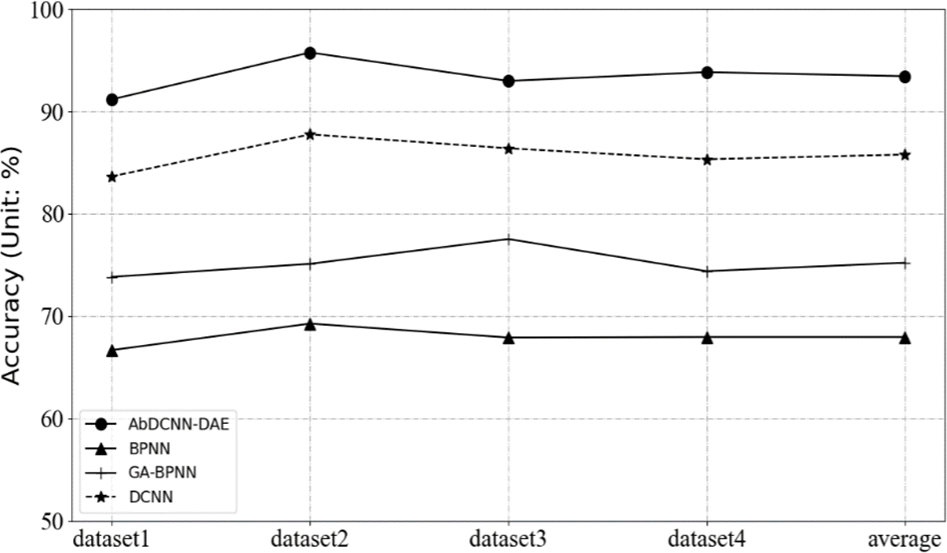Fig. 2. Comparison results of different algorithms on different datasets.

The Fig. 3 shows the Precision, Recall and F1-Score values of the four algorithms after evaluation on the full dataset. Among them, the Precision, Recall and F1-Score values of the algorithm proposed in this paper are: 94.41%, 94.64%, and 94.52%, respectively, which are significantly higher than the other three different algorithms. The Precision, Recall and F1-score values of the DCNN algorithm are: 85.83%, 85.68%, and 85.75%. The experimental results of GA-BPNN algorithm and BPNN algorithm are the worst, the results are: 75.13%, 74.34%, 74.73% and 67.24%, 67.11%, 67.17% respectively.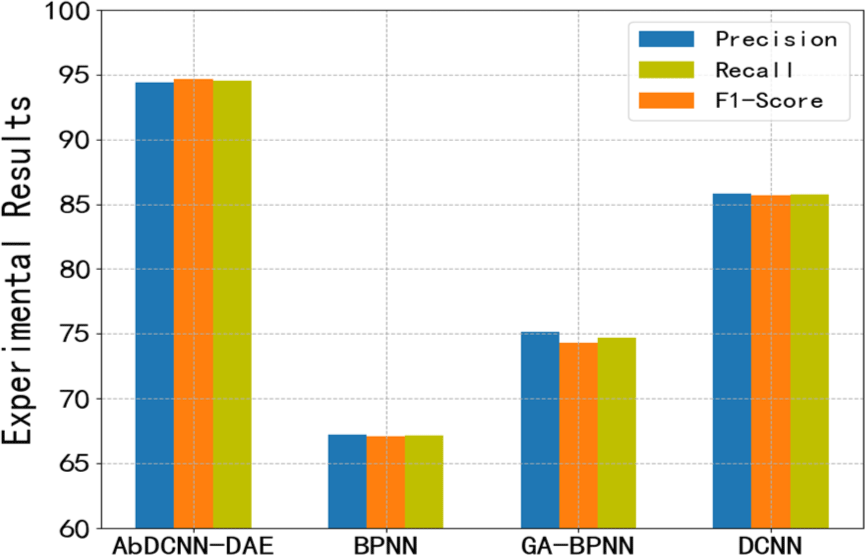Fig. 3. Experimental results on precision, recall and F1-score.

## V. CONCLUSION

The data from energy storage power stations forms the foundation and prerequisite for ensuring successful "multi-station integration." However, as the development scale of energy storage power stations in my country continues to expand, the quantity and variety of data generated by these power stations have witnessed an exponential growth trend. The data generated by energy storage power stations are characterized by their heterogeneity and large-scale nature. An effective data fusion algorithm can integrate the vast amount of heterogeneous data from multiple sources of energy storage power stations. This integration facilitates the interconnection, exchange, and sharing of data between energy storage power stations and other components of the power system. This can help realize the collaboration of various enterprises and systems, and fully explore the potential value of electric power big data. This paper proposes an AbDCNN-DAE algorithm that combines a noise reduction encoder and an attention mechanism model. The DAE network preprocesses and restores the original data to enhance the robustness of the features. The introduction of the self-attention mechanism module improves the ability to capture long-distance temporal correlation of time series data to a certain extent. The experimental results demonstrate that the AbDCNN-DAE algorithm exhibits excellent performance.

## ACKNOWLEDGMENTS

This work was supported by the Technology Project of State Grid Co., Ltd. (under Granted No. B311JP220003)

## REFERENCES

.

D. Muirhead, M. A. Imran, and K. Arshad, "A survey of the challenges, opportunities and use of multiple antennas in current and future 5G small cell base stations," IEEE Access, vol. 4, pp. 2952-2964, 2016..

Y. Yang, W. Gao, S. Guo, Y. Mao, and Y. Yang, "Introduction to BeiDou‐3 navigation satellite system," Navigation, vol. 66, no. 1, pp. 7-18, 2019..

A. Mirzabaev, A. J. Isakov, S. Mirzabekov, T. Makhkamov, and D. Kodirov, "Problems of integration of the photovoltaic power stations with the grid systems," in IOP Conference Series: Earth and Environmental Science, Dec. 2020, vol. 614, no. 1, p. 012016..

J. Chongwatpol, "Managing big data in coal-fired power plants: A business intelligence framework," Industrial Management & Data Systems, 2016..

A. Majumder, L. Behera, and V. K. Subramanian, "Automatic facial expression recognition system using deep network-based data fusion," IEEE Transactions on Cybernetics, vol. 48, no. 1, pp. 103-114, 2016.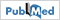.

Y. Gu, H. Yan, X. Zhang, Z. Liu, and F. Ren, "3-D facial expression recognition via attention-based multichannel data fusion network," IEEE Transactions on Instrumentation and Measurement, vol. 70, pp. 1-10, 2021..

C. Zhao, C. R. Tang, and S. Wan, "Multisensor information fusion based on DS evidence theory and BP neural network," in Key Engineering Materials, 2013, vol. 567, pp. 113-117..

K. Ma, H. Zhang, R. Wang, and Z. Zhang, "Target tracking system for multi-sensor data fusion," in 2017 IEEE 2nd Information Technology, Networking, Electronic and Automation Control Conference (ITNEC), Dec. 2017, pp. 1768-1772..

T. C. Fu, "A review on time series data mining," Engineering Applications of Artificial Intelligence, vol. 24, no. 1, pp. 164-181, 2011..

P. Esling and C. Agon, "Time-series data mining," ACM Computing Surveys (CSUR), vol. 45, no. 1, pp. 1-34, 2012..

X. Tao, D. Kong, Y. Wei, and Y. Wang, "A big network traffic data fusion approach based on fisher and deep auto-encoder," Information, vol. 7, no. 2, p. 20, 2016..

A. Noureldin, R. Sharaf, A. Osman, and N. El-Sheimy, "INS/GPS data fusion technique utilizing radial basis functions neural networks," in PLANS 2004. Position Location and Navigation Symposium, Apr. 2004, pp. 280-284..

Y. W. Li and K. Cao, "Establishment and application of intelligent city building information model based on BP neural network model," Computer Communications, vol. 153, pp. 382-389, 2020..

L. Wu, L. Chen, and X. Hao, "Multi-sensor data fusion algorithm for indoor fire early warning based on BP neural network," Information, vol. 12, no. 2, p. 59, 2021..

S. Dabetwar, S. Ekwaro-Osire, and J. P. Dias, "Damage detection of composite materials using data fusion with deep neural networks," in Turbo Expo: Power for Land, Sea, and Air, Sep. 2020, vol. 84225, p. V10-BT27A019..

S. Li, H. Wang, L. Song, P. Wang, L. Cui, and T. Lin, "An adaptive data fusion strategy for fault diagnosis based on the convolutional neural network," Measurement, vol. 165, p. 108122, 2020..

M. A. Nielsen, Neural Networks and Deep Learning. San Francisco, CA: Determination press, 2015, vol. 25..

P. Fergus, C. Chalmers, C. C. Montanez, D. Reilly, P. Lisboa, and B. Pineles, "Modelling segmented cardiotocography time-series signals using one-dimensional convolutional neural networks for the early detection of abnormal birth outcomes," IEEE Transactions on Emerging Topics in Computational Intelligence, vol. 5, no. 6, pp. 882-892, 2020..

L. Jing, T. Wang, M. Zhao, and P. Wang, "An adaptive multi-sensor data fusion method based on deep convolutional neural networks for fault diagnosis of planetary gearbox," Sensors, vol. 17, vol. 2, pp. 414, 2017..

H. T. Chiang, Y. Y. Hsieh, S. W. Fu, K. H. Hung, Y. Tsao, and S. Y. Chien, "Noise reduction in ECG signals using fully convolutional denoising autoencoders," IEEE Access, vol. 7, p. 60806-60813, 2019..

A. Roy, M. Saffar, A. Vaswani, and D. Grangier, "Efficient content-based sparse attention with routing transformers," Transactions of the Association for Computational Linguistics, vol. 9, pp. 53-68, 2021..

K. Cho, A. Courville, and Y. Bengio, "Describing multimedia content using attention-based encoder-decoder networks," IEEE Transactions on Multimedia, vol. 17, no. 11, pp. 1875-1886, 2015..

X. Wu, G. Jiang, X. Wang, P. Xie, and X. Li, "A multi-level-denoising autoencoder approach for wind turbine fault detection," IEEE Access, vol. 7, pp. 59376-59387, 2019..

S. Harbola and V. Coors, "One dimensional convolutional neural network architectures for wind prediction," Energy Conversion and Management, vol. 195, pp. 70-75, 2019..

J. Bouvrie, "Notes on convolutional neural networks," 2006..

J. Zhou, A. H. Gandomi, F. Chen, and A. Holzinger, "Evaluating the quality of machine learning explanations: A survey on methods and metrics," Electronics, vol. 10, no. 5, p. 593, 2021.## AUTHORS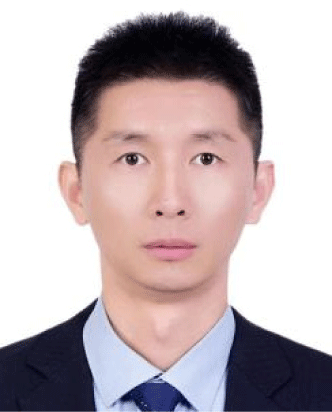Yimin Deng is a senior engineer. He is currently working at State Grid Zhejiang Electric Power Co., Ltd. His research interests include power transmission and transformation, research development, etc.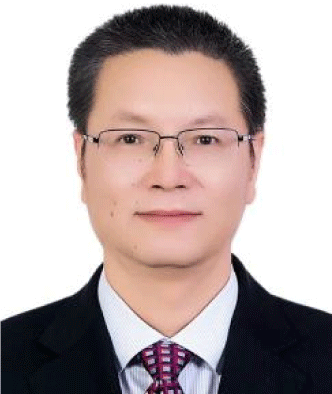Zhoubo Weng is a senior engineer. He is currently working at State Grid Zhejiang Electric Power Co., Ltd. His research interests include electrical power system, scientific research management, etc.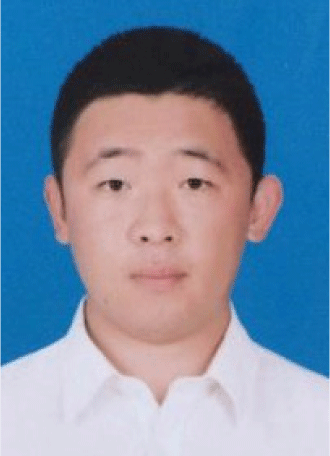Tianlong Zhang is a senior engineer. He is currently working at State Grid Zhe-jiang Electric Power Co., Ltd. His research interests include energy storage power station operation and maintenance.